Showing first {{hits.length}} results of {{hits_total}} for {{searchQueryText}}{{hits.length}} results for {{searchQueryText}}

검색 결과 없음

Contents 1 Integrals 2 Multiple integrals 3 Sums and products 4 Limits 5 Examples 6 Further reading Integrals Integral expression can be added using the \int_{lower}^{upper} command. Note, that integral expression may seems a little different in inline and display math mode. LaTeX code Output Integral $$\int_{a}^{b} x^2 \,dx$$ inside text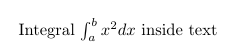$\int_{a}^{b} x^2 \,dx$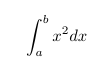Multiple integrals To obtain double/triple/multiple integrals and cyclic integrals you must use amsmath and esint (for cyclic integrals) packages. LaTeX code Output \begin{gather*} \iint_V \mu(u,v) \,du\,dv \\ \iiint_V \mu(u,v,w) \,du\,dv\,dw \\ \iiiint_V \mu(t,u,v,w) \,dt\,du\,dv\,dw \\ \idotsint_V \mu(u_1,\dots,u_k) \,du_1 \dots du_k \end{gather*}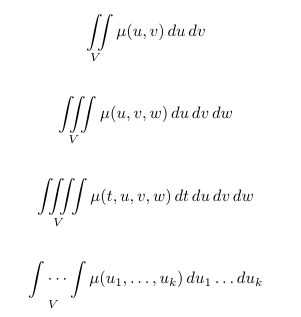$\oint_V f(s) \,ds$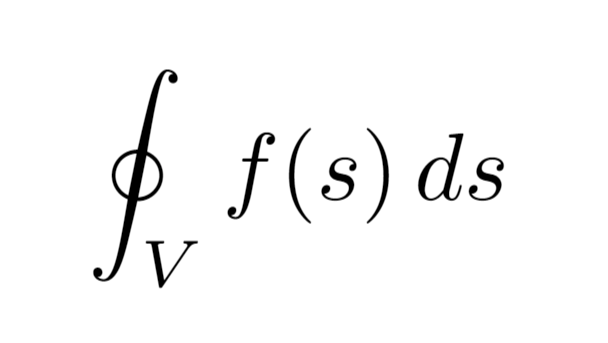Sums and products Like integral, sum expression can be added using the \sum_{lower}^{upper} command. LaTeX code Output Sum $\sum_{n=1}^{\infty} 2^{-n} = 1$ inside text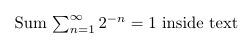$\sum_{n=1}^{\infty} 2^{-n} = 1$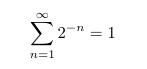In similar way you can obtain expression with product of a sequence of factors using the \prod_{lower}^{upper} command. LaTeX code Output Product $\prod_{i=a}^{b} f(i)$ inside text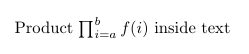$\prod_{i=a}^{b} f(i)$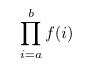Limits Limit expression can be added using the \lim_{lower} command. LaTeX code Output Limit $\lim_{x\to\infty} f(x)$ inside text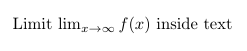$\lim_{x\to\infty} f(x)$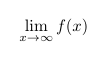Examples Here is an example LaTeX document: \documentclass{article} \title{Integrals, Sums and Limits} \author{Overleaf} \date{} \usepackage{amsmath} \begin{document} \maketitle \section{Integrals} Integral $$\int_{a}^{b} x^2 dx$$ inside text. \medskip The same integral on display: $\int_{a}^{b} x^2 \,dx$ and multiple integrals: \begin{gather*} \iint_V \mu(u,v) \,du\,dv \\ \iiint_V \mu(u,v,w) \,du\,dv\,dw \\ \iiiint_V \mu(t,u,v,w) \,dt\,du\,dv\,dw \\ \idotsint_V \mu(u_1,\dots,u_k) \,du_1 \dots du_k \\ \oint_V f(s) \,ds \end{gather*} \section{Sums and products} Sum $$\sum_{n=1}^{\infty} 2^{-n} = 1$$ inside text. The same sum on display: $\sum_{n=1}^{\infty} 2^{-n} = 1$ Product $$\prod_{i=a}^{b} f(i)$$ inside text. The same product on display: $\prod_{i=a}^{b} f(i)$ \section{Limits} Limit $$\lim_{x\to\infty} f(x)$$ inside text. The same limit on display: $\lim_{x\to\infty} f(x)$ \end{document}  Open this example in Overleaf Further reading For more information see Mathematical expressions Subscripts and superscripts Fractions and Binomials Display style in math mode